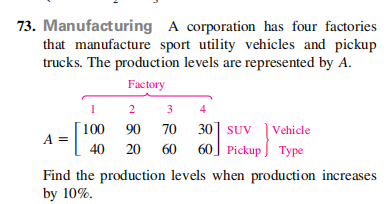### ¿Todavía tienes preguntas de matemáticas?

Pregunte a nuestros tutores expertos
Algebra
Pregunta

A corporation has four factories that manufacture sport utility vehicles and pickup trucks. The production levels are represented by $$A$$ .

$$A = \left[ \begin{array} { r r r r } { 100 } & { 90 } & { 70 } & { 30 } \\ { 40 } & { 20 } & { 60 } & { 60 } \end{array} \right]$$

Find the production levels when production increases by $$10 \%$$ .A = $$\begin{bmatrix}110&99&77&33\\44&22&66&66\\\end{bmatrix}$$